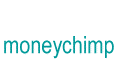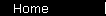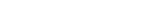# Present Value and CAGR Formula

PV   =   FV / (1 + r)Y

CAGR   =   (FV / PV)1 / Y  -  1

where PV is the present value (= starting principal), FV is the future value, r and CAGR are the annual interest rate, and Y is the number of years invested.

## Understanding the Formulas

Present Value is like Future Value in reverse: you assume you already know the future value of your investment, and want to know what your starting principal will have to be in order to reach your goal in the desired amount of time.The formula for present value is simple; just take the formula for future value and solve for starting principal:

 1 PV   =   FV / (1 + r)Y

(We're now writing PV, for "present value", where we were writing P before. This is sort of the convention. It's still the same quantity: it's the principal you start out with.)

Sometimes people like to assume that they know both the future value and the present value, and they want to find the interest rate required to make it happen. Again the formula is simple: solve the future value formula for r:

 2 r   =   (FV / PV)1 / Y  -  1

The interest rate is often called the "discount rate" when it's the thing you're solving for, and you're assuming that the future value is a given. It's also known as the "internal rate of return", the "equivalent rate of return", or the CAGR (for Compound Annual Growth Rate).

Solving for either the present value or the interest rate may seem like a pretty backwards way of doing things, but these are very useful techniques. They're especially nice for comparing all kinds of different strange investments, by making them all look like ordinary compound interest problems. For example:

Example:   Suppose you have \$1000 to invest, and two characters from down at the barbershop have offered to cut you in on their private money-making schemes. Peter promises to triple your money in five years. Warren says he'll quadruple your money in seven years. Assuming that you are a big enough twit to believe either one of these mendacious scoundrels, which is the better deal?

To find the answer, just use equation 2 (or the popup calculator) to get the rate of return for both investments.

 Peter Warren PV \$1000 \$1000 FV \$3000 \$4000 Y 5 7 r 24.57% 21.90%

Another Example:   Abby promises to pay you \$5000 in five years. How much should you pay her now for the privilege?

This one is more interesting: it's a present value problem, but you yourself have to decide what discount rate to use. We'll assume you think Abby is about as risky as the stock market, and use a discount rate of 11%, about what the annualized market return has been over the past many decades. (That's a number we use throughout the site; other people will recommend using a different number.) So you use equation 1, and get:

PV = \$5000 / (1 + 0.11)5 = \$2,967

meaning \$2,967 is the maximum amount you should be willing to pay her now for her promise of \$5,000 later.

These two examples illustrate the two techniques that are used to perform all valuation problems. You find the equivalent rate of return when you want to know the yield to maturity of a bond at a given market price; and you calculate the present values of future company earnings when you want to know the fair value of a share of stock.

home  |  article  |  glossary  |  calculator  |  about us  |  booksIntroductionCompound InterestMore CompoundingPresent Value / CAGRComposite InvestmentsBond YieldGeometric SeriesGrowth + ContributionsAnnuityMortgageStock ValuationBooks & Links

 Also see the present value and return rate calculators.